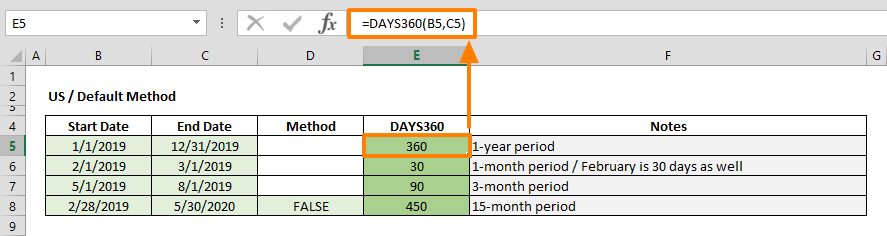The DAYS360 function is a Date & Time formula that calculates and returns the number of days between two given dates, based on a 360-day year (12 x 30 days per month). 360-day year base is used in some accounting calculations which assumes that 1-year period is 360 days. In this guide, we’re going to show you how to use the Excel DAYS360 function and also go over some tips and error handling methods.

## Supported versions

• All Excel versions

## DAYS360 Function Syntax

DAYS360(start_date, end_date, [method])

## Arguments

 start_date Start date of the period you want to get days between. Microsoft recommends using the DATE or other functions that return a date serial number. end_date End date of the period you want to get days between. Microsoft recommends using the DATE or other functions that return a date serial number. [method] Optional. A Boolean value that specifies the method of day count. FALSE: US (Default value) TRUE: European

### Method

 Method Defined FALSE or omitted U.S. (NASD) method. If the starting date is the last day of a month, the function sets it to the 30th day of the same month. If the ending date is the last day of a month: If the starting date is earlier than the 30th day of a month, the function sets the ending date to the 1st day of the next month. Otherwise the ending date is set to the 30th day of the same month. TRUE European method. Starting dates and ending dates that occur on the 31st day of a month are set to the 30th day of the same month.

## Examples

### US or default method

To calculate number of days with the US method, enter a value for the start_date and end_date arguments. Microsoft recommends using functions to generate dates as serial numbers. Thus, you can use the DATE function to generate the date you desire. Static dates typed in a cell will also work.

Because of the 360-day year concept, some calculations may return different values than using a 365-day year. For example, there are 360 days between 1/1/2019 and 12/31/2019:

=DAYS360(DATE(2019,1,1),DATE(2019,12,31)) returns 360

Similarly, the DAYS360 function calculates February as 30 days:

=DAYS360(DATE(2019,2,1),DATE(2019,3,1)) returns 30

Alternatively, you can enter FALSE for the [method] argument to calculate using the US type.

=DAYS360(DATE(2019,2,28),DATE(2020,5,30),FALSE) returns 450### European Method

European method sets the starting date and end date to the 30th day of the same month, if they occur on the 31st day of a month. This approach may cause different outcomes in respect to the default (US) method.

For example, while the US method calculates 30 days between Jan 1st and Jan 31st, European method returns 29 days because it sets the 31st day to 30th.

=DAYS360(DATE(2019,1,1),DATE(2019,1,31),TRUE)## Tips

• DAYS360 function ignores time value of dates.
• Excel keeps date and time values as numbers. Excel assumes that Jan 1st, 1900 is 1, and every subsequent date value is based on this. While whole numbers represent days, decimals represent time values. For example; 1/1/2018 is equal to 43101, and 12:00 is equal to 5.

## Issues

• If text arguments are not valid dates, the DAYS360 function returns #VALUE! error value. Use the DATE function to avoid this.